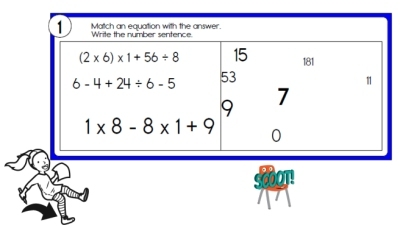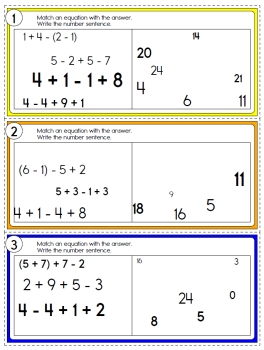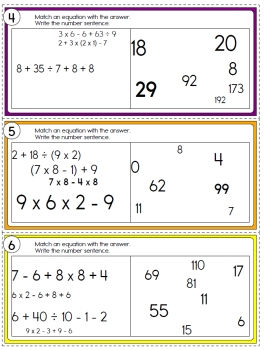Worksheets and No Prep Teaching Resources
Scoot Games
Scoot Math
Order of Operations

Scoot Order of Operations Math GamesOrder of Operations Math Scoot - Easier: Just Addition and Subtraction Order of Operations Math Scoot (3 numbers in each equation) Order of Operations Math Scoot (3-4 numbers in each equation) Order of Operations Math Scoot (3-5 numbers in each equation)Order of Operations Math Scoot - Addition, Subtraction, Multiplication, and Division Order of Operations Math Scoot (3 numbers in each equation) Order of Operations Math Scoot (3-4 numbers in each equation) Order of Operations Math Scoot (3-5 numbers in each equation)